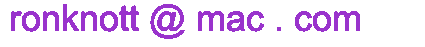## The Natural Number String 0123456789101112131415... BETA Test 5 Jan 2017

The Natural Number String is the infinite string 0123456789101112131415... made by joining together all the natural numbers.
Every number will eventually appear in the string of course, but some appear earlier than expected, such as "12" which is between "0" and "3" as well as its natural place between "11" and "13". Here are some other mathematical investigations at school level (pre-university).
The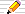icon means there is a You Do The Maths... section of questions to start your own investigations.
The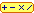calculator icon indicates that there is a live interactive calculator in that section.

## The Early Bird Numbers

What digit is at position i if the positions start at 0 for number 0 and what number is the digit in?
For example, the digit at position 20 is the "1" in number 15
 position number 0 1 2 3 4 5 6 7 8 9 10 11 12 13 14 15 16 17 18 19 20 21 ... 188 189 ... 0 1 2 3 4 5 6 7 8 9 10 11 12 13 14 15 ... 99 ...

Each position (index number) in the string will have in it a particular digit of one of the natural numbers that were joined together. So number 12 in the sequence is in positions 14, 15 and at position 15 is a digit of the number 12. As a string, "12" appears in many places in the sequence.
Where is the first appearance of number N?
For example 12 appears at positions which is before its "natural" place in the sequence, at ... and so 12 is called an Early Bird number.
We separate a sequence's natural numbers by using '.
Find all the positions at which a string occurs.
For example:
up to position 99 (in number 54) '0' occurs 6 times at positions : 0, 11, 31, 51, 71, 91 in numbers: 0, 10, 20, 30, 40, 50.
Count the frequencies of the digits in any range of numbers
For example, the digit counts in all two digit numbers are

###You Do The Maths...

1. 12 is the first Early bird number as it first appears in positions 1,2 in the string. Can you find another?
2. Find all 10 early Bird numbers less than 50
1. Why are all the numbers 91, 92, 93, 94, 95, 96, 97, 98, 99 Early Bird numbers?
2. Are 90 and 100 Early Birds?

## Plots

Here is some plots of
• the first position (y-axis) of each number (x-axis).
The natural position of each in the topmost sloping line and any points below this indicate that the x-number is an Early Bird.
• all the early positions of a number, that is all the starting positions of the number string up to its natural position in the sequence.
• all positions of a number both before and after its natural position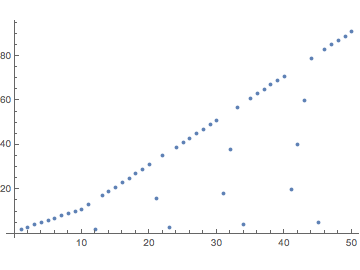First positions: 0-50 0-100 0-500 0-1000 0-2000 0-3000 0-5000 0-10000 0-50 0-100 0-500 0-1000 0-2000 0-3000 0-5000 0-10000 0-50 0-100 0-500 0-1000 0-2000 0-3000 0-5000 0-10000

##The Natural Number Sequence Calculator

Base
set the number base for all parts of the Calculator. The usual base is 10 but it can vary from 2 to 36. Use digits 0 1 2 3 4 5 6 7 8 9 a b c d e f ... x y z, upper or lower case.
The positions input
are given as ordinary (base 10) numbers always and start with number 0 in position 0 (at index number 0).
They can be arbitrarily large!
For example:
In the (base 10) sequence, the 10 000 000 000 000 000 000 000-th digit (10^22 or the ten billion trillion-th) is the middle 4 in the number 481481481481481481481
Find number(s) in position(s):
What numbers are in the given positions (index numbers) is the natural-sequence-string for the given number Base at the top?
To give a single position leave the 'up to' input empty.
Search for a digit string in the given positions
The digit string can be a number or other string of digits beginning with 0 such as '012' or '00'. Remember that the digits must be valid in the Base at the top of the Calculator.
For the numbers in the range on the right (or leave 'up to' blank for just a single number),
which can be given as ordinary (base 10) numbers or their Base equivalent.
Some calculations allow arbitrarily large numbers but others need smaller integers in this version of the calculator as they perform a 'brute force' search.
[Restricted numbers] means:
The Calculator may have to search up to the 'natural position' of the numbers and this is not recommended for a search involving more than
Find [the first position]
just one position is returned, which is always less than or equal to the natural position.
[Restricted numbers]
Find [the natural position]
is the position the number occurs in the natural sequence order, that is, as a single number n with n-1 before it and n+1 after.
The number(s) can be arbitrarily large! For example:
The number 12345679012345678901234567890 is at 'natural position' 346913580246913577024691357700..346913580246913577024691357728.
Find [all early positions]
indicates all the positions the number is found in are reported, up to and including the natural position of the number(s). [Restricted numbers]
Count [EarlyBirds, non-Early Birds]
Using the given number range all the (non-) Early Birds are found and the total number reported. [Restricted numbers]
Show [EarlyBirds, non-Early Birds]
the list of all qualifying numbers is counted and output too. [Restricted numbers]
Count digit frequencies
the frequency of each digit for the given Base is found and counted using all the numbers in the specified number range.
The numbers can be arbitrarily large.
Convert a number to/from base 10
give a base 10 number to convert TO the Base at the top of the Calculator or
give a valid number in the Base at the top to convert to base 10.
The number to convert can be an arbitrarily large!
C A L C U L A T O R
Natural Number String in base
 number(s) in position up to for digit string the first position the natural position all early positions of number base 10 in base up to Early Birds non-Early Birds in range digit frequencies in from base 10 to base 10

R E S U L T S

###You Do The Maths...

1. How many digits are there in total in the two digit numbers 10 to 99?
2. How many digits are there in total in the three digit numbers 100 to 999?
3. Find a formula for:
1. the first n-digit number
2. the last n-digit number
3. how total number of digits in all the n-digit numbers
4. Following on from the previous investigation, find a formula or else a method of computing the starting position of the n-digit numbers in the (base 10) natural sequence.
1. Using your answer to the previous investigation, find a method of calculating the natural position of the number N in the sequence.
1. Find the 3 places that 121 occurs up to and including its natural position: 253-255.
2. Find the 5 places that 1211 occurs up to and including its natural position: 3734-3737.
3. Find the 7 places that 12111 occurs up to and including its natural position: 49445-49449.
2. Looking at the Plots above, what can you say about the numbers 90-100, 900-1000 and 9000-10000?
Check your answer using   in range  in the Calculator for each of these ranges.
3. Using   in range   in the Calculator, investigate the proportion of numbers which are Early Birds as you go further and further along the sequence.
Does it look as if the proportion grows or decreases as we go deeper into the sequence?
4. As the numbers get larger, there are longer and longer runs of Early Bird numbers. For instance,
• all 14 numbers in the range from 210 to 223 are Early Birds
• all 100 in the range from 901 to 999
• all 457 in the range 5100 to 5556
• all 910 in the range 9091 to 9999!
Can you identify more of these ranges?
1. What can you say about the numbers in this series: 90, 900, 9000, ...?
2. What can you say about the numbers in this series: 91, 901, 90001, ...?
1. are there an infinite number of Early Birds?
2. are there an infinite number of non-Early Birds?
5. Look at which numbers contain the 10th, 100th, 1000th, ... etc digit in the base 10 sequence.
What patterns or special numbers do you spot there?
Can you explain any of the patterns mathematically?
6. (Hard!) The longest runs of non-Early Bird (base 10) numbers seem to be of length 9, for instance 1001 and 1011 are Early Birds but all 9 number between them are not. Is a longer run possible? Why?
7. (Hard!) Using the Calculator above to check your answer, find a method of computing all the positions at which a given number string appears in the sequence.
8. (Hard!) Using the Calculator above to check your answer, find a method of computing the position at which a given number (string of digits) first appears in the sequence.

### The Binary Number String: 011011100101110...

If we wrote the natural numbers in base 2 (binary), we have
 position Binary n 0 1 2 3 4 5 6 7 8 9 10 11 12 13 14 15 16 17 18 19 20 21 22 ... 0 1 1 0 1 1 1 0 0 1 0 1 1 1 0 1 1 1 1 0 0 0 ... 0 1 2 3 4 5 6 7 8 ...
Again there is a "natural position" for every binary number n and some binary number strings (bit patterns) appear before their natural position,such as 3=112 which is in positions 1 and 2 as well as its natural place in positions 4-5.

###You Do The Maths...

1. Number 3=112 is the first binary Early bird number. What is the next number?
2. Find all 10 binary Early Bird numbers less than 16.

### Binary Plots

As for the decimal number plots above, here are the plots of the positions (y-axis) of the first occurrences of each binary number (x-axis).
The natural position of each in the topmost sloping line and any points below this indicate that the x-number is a binary Early Bird.
You can also compare these with a plot of all the binary early positions of a number, that is all the staring positions of the binary number string up to its natural position in the binary sequence.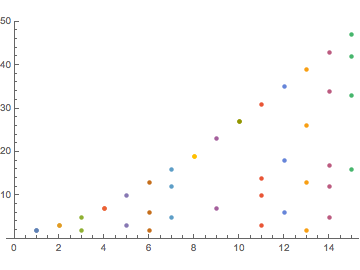First positions: 0-15 0-32 0-64 0-128 0-256 0-15 0-32 0-64 0-128 0-256 0-15 0-32 0-64 0-128 0-256

###You Do The Maths...

1. Which Early Birds start at position 1?
In other words, if we take the base B natural sequence and ignore the initial zero, by taking the first n digits we have a base B number which must be an Early Bird. What is the sequence for base B?
For base 10, it is 1, 12, 123, 1234, … , 1234567891, 12345678910, … but for base 2 it is
1, 11, 110, 1101, 11011, 110111, 1101110, … and as decimal numbers these are
1, 3, 6, 13, 27, 55, 110, … (A055143), all the base 2 Early Bird numbers whose first appearance is at position 1.
What are the Early Birds in base 3 that start at position 1?
What about base 4? base 5?
Check your answers with base 3:A055144, base 4:A055145, base 5:A055146, base 6:A055147, base 7:A055148, base 8:A055149, base 9:A055150, base 10:A252043.

## The decimal number 0.12345678910111213 ...

If we make our infinite number string into a decimal number by putting a decimal point at the start, we can ask some interesting (mathematical) questions about it.

### Is it rational (a fraction)?

No, because all rational numbers when written as a decimal will either stop (such as 3/8=0.375) or else end up repeating the same digits in a cycle for ever (such as 1/3=0.33333... or 2/7=0.285714 285714...). Our decimal does not terminate and neither does it end in the same cycle repeating for ever.
Our decimal is therefore of a irrational number - one that cannot be written exactly as a fraction.

### Is it like √2, the root of a polynomial?

The square-root of 2 is famously irrational but we can describe it as
• the positive solution to the equation x2 = 2 or as
• the positive root of the polynomial x2 − 2
A polynomial in x is a finite sum of whole number multiples of powers of x such as 5 − 4 x3 or 1 + 2x3 − 3x3 + 7x10.

A root of a polynomial in x is any value for x that makes the polynomial's value 0.
All rational numbers are the roots of a simple polynomial since a/b is the root of b x − a. Similarly, all square-roots √a are - as their name implies - roots of a square polynomial x2 − a.

If a number is the root of some polynomial, it is called an algebraic number
But some numbers, such as π and e and loge(2) are neither rational nor algebraic.
Numbers that are not algebraic are called transcendental. If they are a root of a polynomial then it must be an infinite polynomial, also called a power series.
The French mathematician Joseph Liouville (1809-1882) was the first person to prove that there are numbers that are transcendental.
It seems to be quite difficult to prove a number is transcendental!

However, our decimal 0.12345678910111213... was proved transcendental in a paper in German published in 1937 by Kurt Mahler (1903-1988).
It is sometimes called Champernowne's Number because a few years earlier D G Champernowne had proved that the number is normal in the sense that each digit appears 1/10 of the time in the long run when written as a decimal.

• Über die Dezimalbruchentwicklung gewisser Irrationalzahlen K Mahler, Mathematica B (Holland, 1937-1938), pages 2-16 is the paper in German where Mahler proves 0.123456789101112... is transcendental.
• The construction of decimals normal in the scale of ten D G Champernowne, Journal of London Math Soc vol 52 (1933), pages 254-260 is where he shows 0.123456789101112... is normal, that is, that each of the digits 0 to 9 is 'equally distributed' (appear 1/10 of the time when taken to the limit).
You might think that "each digit appears with the same frequency on average" is a condition for a number to be "random". But for 0.123456789101112... even though the digits are uniformly distributed (each occurs with the same probability in the entire number) it appears to be far from our idea of a "random number" when we write it down!

### Champernowne Numbers in different bases

If we concatenate the base 2 numbers we get the base 2 Champernowne Number:
binary Champernowne number: 0.1 10 11 100 101 110 111 1000 ...2
which, as with the other Champernowne numbers to any base, is irrational (not a fraction).
The base 4 value has a remarkable value when written as an ordinary decimal fraction:
base 4 Champernowne number: 0.1 2 3 10 11 12 13 20 21 22 23 ... 33 100 101 ...4 = 0.4261111111111110657645566
The decimal value 0.4261111... = 0.4261 is the fraction 297/697 so this is an excellent approximation to the base 4 Champernowne number!
And - would you believe it for there seems no simple or obvious mathematical reason why - the same thing happens with base 5:
base 5 Champernowne Number: 0.1 2 3 4 10 11 12 13 14 20 21... 44 100 101...5 = 0.31073611111111111111111111111096303...
0.3107361111... = 0.3107361 = 22373/72000
= 0.1234101112131420212223243031323334404142440001...5
and is accurate to 41 base 5 places, the start of the natural position of 23 = 435.
This simple kind of pattern is not repeated for bases 2 and 3 nor for bases 6, 7, 8 however - at least not for base 10 decimals. But if we are looking for simple patterns in the decimal expansion, we are overlooking some cases where the decimal pattern is not initially obvious, as for example in 1/7 = 0. 142859 142859 ... and do we know the expansions in other bases? Perhaps there are more patterns in other bases?
There are more facts, figures and Calculators to explore the many patterns on the Decimal Fractions page at this site.

A much easier way to spot these patterns is to be mathematical and use Continued Fractions, and this we begin to explore in the next section.

To find the best fractions approximating a number, we use Continued Fractions. There is an Introduction to Continued Fractions page on this site if you have not met these simple extended fractions before.

### Champernowne Numbers and Continued Fractions

For our decimal Champernowne number at the start of this section, its continued fraction (CF) is
0.12345678910111213... = [0; 8, 9, 1, 149083, 1, 1, 1, 4, 1, 1, 1, 3, ...]
The presence of the very large term 149083 in its CF shows that the previous convergent will give a remarkably accurate approximation:
convergents: 0, 1/8, 9/73, 10/81, 1490839/12075796, ...
1490839/12075796 = 0.123456789101108...
If we continue with the CF, we find
0.12345678910111213... = [0; 8, 9, 1, 149083, 1, 1, 1, 4, 1, 1, 1, 3, 4, 1, 1, 1, 15, 4575401113910310764836466282429561185996039397104575550006620043930902626592563149379532077471286563138641209375503552094607183089984575801469863148833592141783010987, 6, 1, 1, 21, 1, 9, 1, 1, ...]
That 19-th element has 166 digits! The CF continues with small numbers (no more than 3 digits) until the 41-st which has 2504 digits!
The number of digits in each CF term is given by 0, 1, 1, 1, 6, 1, 1, 1, 1, 1, 1, 1, 1, 1, 1, 1, 1, 2, 166, ... A143532. The astonishingly large numbers appear at positions 5, 19, 41, 163, ... A038705 and the number of digits in these "high water mark" elements are 6, 166, 2504, 33102, ... A143534.

For the base 2 Champernowne number, we have something similar:

0.110111001011101111000...2 = 0.8622401258680545715577903...
continued fraction: [0; 1, 6, 3, 1, 6, 5, 3, 3, 1, 6, 4, 1, 3, 298, 1, 6, 1, 1, ...]
convergent at 298 term: 474023339/549757921 = 0.86224012586805456869...
and in binary this is accurate to the natural position of 16 = 100002 or up to the 54-th binary place:
474023339/549757921 = 0.110111001011101111000100110101011110011011110111110000011...2
The base 4 and 5 Champernowne numbers from above have these CFs and convergents:
base 4: 0.42611111111111106576455... = [0;2,2,1,7,1,1,2,1,1,1,1,6806293849,1,33,157,1,2,1...]
convergents: 0,1/2,2/5,3/7,23/54,26/61,49/115,124/291,173/406,297/697,470/1103,767/1800, ...
767/1800 = 0.4261

base 5: 0.3107361111111111111111111111109630333... = [0;3,4,1,1,2,2,18,1,20,1302701925685142513155, ...]
convergents: 0,1/3,4/13,5/16,9/29,23/74,55/177,1013/3260,1068/3437,22373/72000, ...
22373/72000 = 0.3107361

Now we can reveal the pattern in base 6:
base 6: 0.2398626858150667674477198286722096245905769715293502137... = [0;4,5,1,10,1,4,3,9,1,2,2,1,1,699745284439054751106354294914368414245,...]
convergents: 0,1/4,5/21,6/25,65/271,71/296,349/1455,1118/4661,10411/43404,11529/48065,33469/139534,78467/327133,111936/466667,190403/793800,133233601393049341774903176814580489177602671/555457806787721661428224039303025647228147667
190403/793800 = 0.23986268581506676744771982867220962459057697152935248173...
This phenomenon continues to all subsequent bases!
For base 7, the amazingly accurate fraction 7529191/38723328 is correct to 77 decimal places; base 8 has 84934399/520224768 with an accuracy of 108 dps and base 9's approximation of 4304671369/30611001600 is correct to 146dps!
To fill in the gaps for bases 2 and 3, we have:
base 2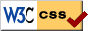© 2016 Dr Ron Knott